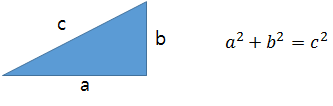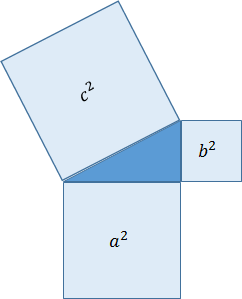# Pythagorean Theorem 2

Pythagorean Theorem

When the three sides of a right triangle are a, b, and c, and the longest side is c, the following equation is always true.The areas of a square with sides a, b, and c is a 2, b2, and c2. Assuming three squares made with each side, the sum of the areas of the two smaller squares equals the larger square.Pythagoras is an ancient Greek philosopher who lived around 500 BC.
According to the records, "Pythagorean Theorem" was not discovered by Pythagoras. Before the birth of Pythagoras, the same concept was already known throughout the world, such as ancient Egypt and China. And it was also used in architecture, surveying, and so on.
Nevertheless, it is said that this concept came from the geometric book written by Euclid in the name of "Pythagoras theorem". The "Pythagorean theorem" is recorded in Euclid 's Principle, which was used in Europe as a mathematics textbook for over 2000 years.

Application of Pythagorean Theorem

The Pythagorean theorem was often used in the process of creating Javalab.
For example, the way to find the distance between two points on the screen is to use Pythagorean theorem.